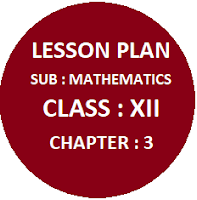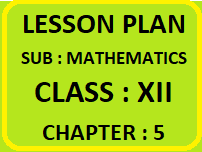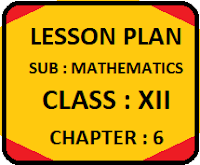### CBSE Assignments class 09 Mathematics

Mathematics Assignments & Worksheets  For  Class IX Chapter-wise mathematics assignment for class 09. Important and useful extra questions strictly according to the CBSE syllabus and pattern with answer key CBSE Mathematics is a very good platform for the students and is contain the assignments for the students from 9 th  to 12 th  standard.  Here students can find very useful content which is very helpful to handle final examinations effectively.  For better understanding of the topic students should revise NCERT book with all examples and then start solving the chapter-wise assignments.  These assignments cover all the topics and are strictly according to the CBSE syllabus.  With the help of these assignments students can easily achieve the examination level and  can reach at the maximum height. Class 09 Mathematics    Assignment Case Study Based Questions Class IX

### Lesson Plan Maths Class XII | For Maths Teacher

Chapter-wise Maths Lesson Plan Class 12

Chapter wise Lesson Plan, Class XII Subject Mathematics for Mathematics Teacher. Effective way of Teaching Mathematics. Top planning by the teacher for effective teaching in the class. E lesson planning for mathematics.

 LESSON PLAN :   CLASS 12BY CBSE MATHEMATICS Chapter 1 Relation & Functions This lesson plan is for the teachers who are teaching mathematics class 10+2 class. LESSON PLAN :   CLASS 12BY CBSE MATHEMATICS Chapter 2 Inverse Trigonometric Functions This lesson plan is for the teachers who are teaching mathematics class 10+2 class. LESSON PLAN :   CLASS 12BY CBSE MATHEMATICS Chapter 3 Metrices This lesson plan is for the teachers who are teaching mathematics class 10+2 class. LESSON PLAN :   CLASS 12BY CBSE MATHEMATICS Chapter 4 Determinants This lesson plan is for the teachers who are teaching mathematics class 10+2 class. LESSON PLAN :   CLASS 12BY CBSE MATHEMATICS Chapter 5 Continuity & Differentiability This lesson plan is for the teachers who are teaching mathematics class 10+2 class. LESSON PLAN :   CLASS 12BY CBSE MATHEMATICS Chapter 6 Applications of Derivatives This lesson plan is for the teachers who are teaching mathematics class 10+2 class. LESSON PLAN :   CLASS 12BY CBSE MATHEMATICS Chapter 7 Integrals This lesson plan is for the teachers who are teaching mathematics class 10+2 class. LESSON PLAN :   CLASS 12BY CBSE MATHEMATICS Chapter 8 Application of Integrals This lesson plan is for the teachers who are teaching mathematics class 10+2 class. LESSON PLAN :   CLASS 12BY CBSE MATHEMATICS Chapter 9 Differential Equations This lesson plan is for the teachers who are teaching mathematics class 10+2 class.

 LESSON PLAN :   CLASS 12 BY CBSE MATHEMATICS Chapter 10 Vector Algebra This lesson plan is for the teachers who are teaching mathematics class 10+2 class. LESSON PLAN :   CLASS 12 BY CBSE MATHEMATICS Chapter 11 Three Dimentional Geometry This lesson plan is for the teachers who are teaching mathematics class 10+2 class. LESSON PLAN :   CLASS 12 BY CBSE MATHEMATICS Chapter 12 Linear Programming This lesson plan is for the teachers who are teaching mathematics class 10+2 class. LESSON PLAN :   CLASS 12BY CBSE MATHEMATICS Chapter 13 Probability This lesson plan is for the teachers who are teaching mathematics class 10+2 classTechniques of Making E-Lesson PlanThis is the technique which every teacher need to make before entering the class room. As every teacher have different method of teaching so that every teacher can have unique lesson plan according to his own strategies. Here I am listing some techniques on the basis of which teacher can make their own lesson plans🙏

1.